# Fluid Mechanics 1

## 20 Questions MCQ Test Mock test series of SSC JE Civil Engineering | Fluid Mechanics 1

Description
This mock test of Fluid Mechanics 1 for Civil Engineering (CE) helps you for every Civil Engineering (CE) entrance exam. This contains 20 Multiple Choice Questions for Civil Engineering (CE) Fluid Mechanics 1 (mcq) to study with solutions a complete question bank. The solved questions answers in this Fluid Mechanics 1 quiz give you a good mix of easy questions and tough questions. Civil Engineering (CE) students definitely take this Fluid Mechanics 1 exercise for a better result in the exam. You can find other Fluid Mechanics 1 extra questions, long questions & short questions for Civil Engineering (CE) on EduRev as well by searching above.
QUESTION: 1

### For Bernoulli’s equation to remain valid, which of the following is NOT required?

Solution:

The conditions to be satisfied for the applicability of Bernoulli’s equation are

I. Flow along a stream line

II. Flow is steady and Incompressible

III. Effect of viscous forces is negligible

QUESTION: 2

### The hydraulic grade line for flow in a pipe of constant internal diameter ______.

Solution:

For the pipe of constant diameter, the hydraulic gradient line is always sloping down in the direction of flow and is parallel to total energy line and the difference between both the lines represents velocity head. Due to hf(head loss due to friction) along the length of pipe, H.G.L always sloping down.

QUESTION: 3

### A hydraulic jump occurs when the grade changes from:

Solution:

When the flow conditions changes form super critical (Fr > 1) to subcritical (Fr < 1), this results to an abrupt rise of water accompanied by turbulent rollers is called as hydraulic Jump or standing wave.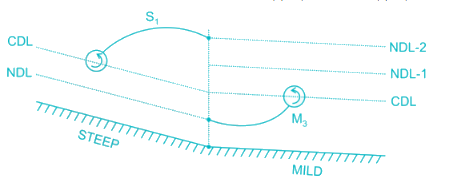The hydraulic Jump occurs when the grade changes from sleep to mild

QUESTION: 4

In an orifice used for flow measurement, the coefficient of velocity is always:

Solution:

Coefficient of velocity through orifice =ActualvelocityTheoreticalvelocity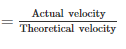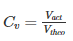Vtheo > Vact

So, Cv is always less than 1.

QUESTION: 5

The centre of pressure on inclined immersed surface remains valid for the centre of pressure on vertical immersed surface, if the angle (θ) at immersed surface with liquid surface is:

Solution:

Centre of Pressure on a Immersed surface from water surface is given by: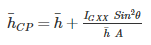For vertical surface θ = 90°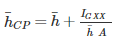So, the formula is valid for θ = 90°

QUESTION: 6

The mechanical efficiency of an impulse turbine is generally between:

Solution:

Mechanical efficiency of Impulse turbine is given by: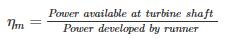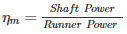It generally varies between 97 to 99

Also, volumetric efficiency (ηv) = 97 to 99

Overall efficiency (ηo) = 85 to 90

QUESTION: 7

Which of the following is CORRECT about the viscosity of gas?

Solution:

As temperature increases, randomness in molecules also increases. This increase in randomness will result in increase in viscosity as viscosity of gas depends on randomness & collision of gas molecules.

Phenomenon: As a gas is heated, the movement of gas molecules increases and the probability that one gas molecule will collide with another gas molecule increases. In other words, increasing gas temperature causes the gas molecules to collide more often. This increases the gas viscosity because the transfer of momentum between stationary and moving molecules is what causes gas viscosity.

QUESTION: 8

Pressure of 200 kPa is equivalent to the head of z metre of liquid having relative density 1.59. The value of z (m) is _____.

Solution:

200 kPa = 200,000 Pa

200,000 Pa = 200,000 N/m²

Pressure in liquids is calculate by the formula:

P = αgh

Where P = pressure, α = density, g = force of gravity,  and h = height

Taking g = 10

αgh = 200,000

1590 x 10 x h = 200,000

h = 200,000/15900

h = 12.58 m

QUESTION: 9

A longitudinal rectangular surface is hanged into the water such that its top and bottom points are at depth of 1.5 m and 6.0 m respectively. The depth of center of pressure (m) from the top surface is _____.

Solution: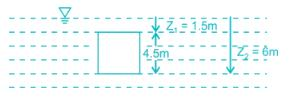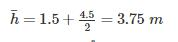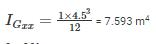θ = 90°

A = 1 × 4.5 m2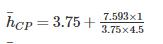h¯CP= 4.2 metre

QUESTION: 10

The velocity potential which follow the equation of continuity is ________

Solution:

Velocity Potential function (ϕ) is given as = x2 - y2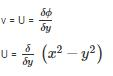u = - 2xV = 2y

For continuity to be satisfied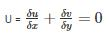-2 + 2 = 0

(Hence satisfied)

QUESTION: 11

The term alternate depths in open channel flow is used to designate the depths

Solution:

For a given discharge Q in a channel, there will be two depths for a given specific energy. These two depths are known as alternate depths.

QUESTION: 12

If a thin plate is held parallel to fluid stream the pressure drag on it is:

Solution:

Viscous forces and pressure forces are responsible for drag forces. There are two types of drag forces present pressure drag and skin friction drag. Pressure drag is due to the wake region behind the body and skin friction drag is due to the viscosity of the fluid.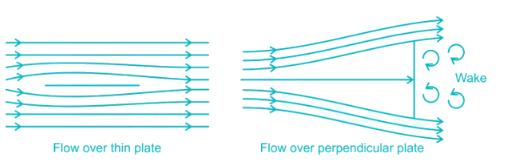In case of flow over a thin plate placed parallel to a stream of flowing fluid, the flow separation (if occurs), would occur only towards the rear end and the wake is negligibly small. The resulting pressure drag will also be very small, approximate to zero.

On the other hand, pressure drag will be large when the thin plate is held perpendicular to the flow.

QUESTION: 13

Two pumps can operate independently at heads H1, H2 and discharges Q1, Q2, respectively. If the pumps are connected in parallel, then what are the resulting discharge (Q) and head (H)?

Solution:

Pumps in Parallel:

Same Head: H = H1 = H2

Add the discharge: Q = Q1 + Q2

Pumps in Series:

Same Discharge: Q = Q1 = Q2

QUESTION: 14

An isentropic process is always...

Solution:

In thermodynamics an isentropic process is an idealized thermodynamic process. that is both adiabatic and reversible. The work transfer of system is friction-less and there is no transfer of heat as matter.

QUESTION: 15

A centrifugal pump delivers a liquid when pressure rise in impeller is equal to _______

Solution:

Manometric head is the head against which head is required to be produced by the pump to deliver water to the destination. Manometric head is higher than the sum of suction and delivery heads because it accounts for head losses due to friction.

QUESTION: 16

Separation of flow occurs when pressure gradient

Solution:

Flow separation occurs when the pressure gradient is positive and velocity gradient is negative.

QUESTION: 17

The most economical section of circular channel for maximum discharge is obtained when (Where, d is the diameter of circular section)

Solution:

D = depth of flow

d = diameter of pipe

Rm = Hydraulic Mean depth = A/P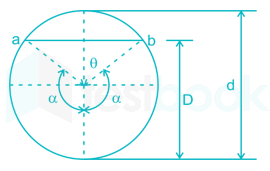Perimeter, P = αd

Condition for Maximum Discharge for Circular Section:

α = 154° = 2.65 radians

D = 0.95 d

Rm = 0.29 d

Condition for Maximum Velocity for Circular Section:

α = 128.75° = 2.25 radians

D = 0.81 d

Rm = 0.3 d

QUESTION: 18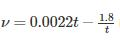is the equation to determine kinematic viscosity of liquids by

Solution:

The device used for measurement of viscosity is known as viscometer. According to Saybolt viscometer: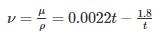QUESTION: 19

A pump delivers water at a rate of 0.025 m3/s against a head of 30 m. If the overall efficiency of the pump is 75%, the power required by the pump is:

Solution: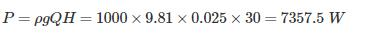Power required: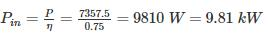QUESTION: 20

A streamlined body is defined as a body about which ________.

Solution:

A stream lined body is defined as the body whose surface coincides with the stream - lines, when the body is placed in a flow. In that case the separation of flow will take place only at trailing edge (or rearmost part of the body).

Behind a stream lined body, wake formation zone will be very small and consequently the pressure drag will be very small. Thus the total drag on the stream lined body will be due to friction (shear) only.